### Beautiful Geometry

What fraction of this large square has been shaded red?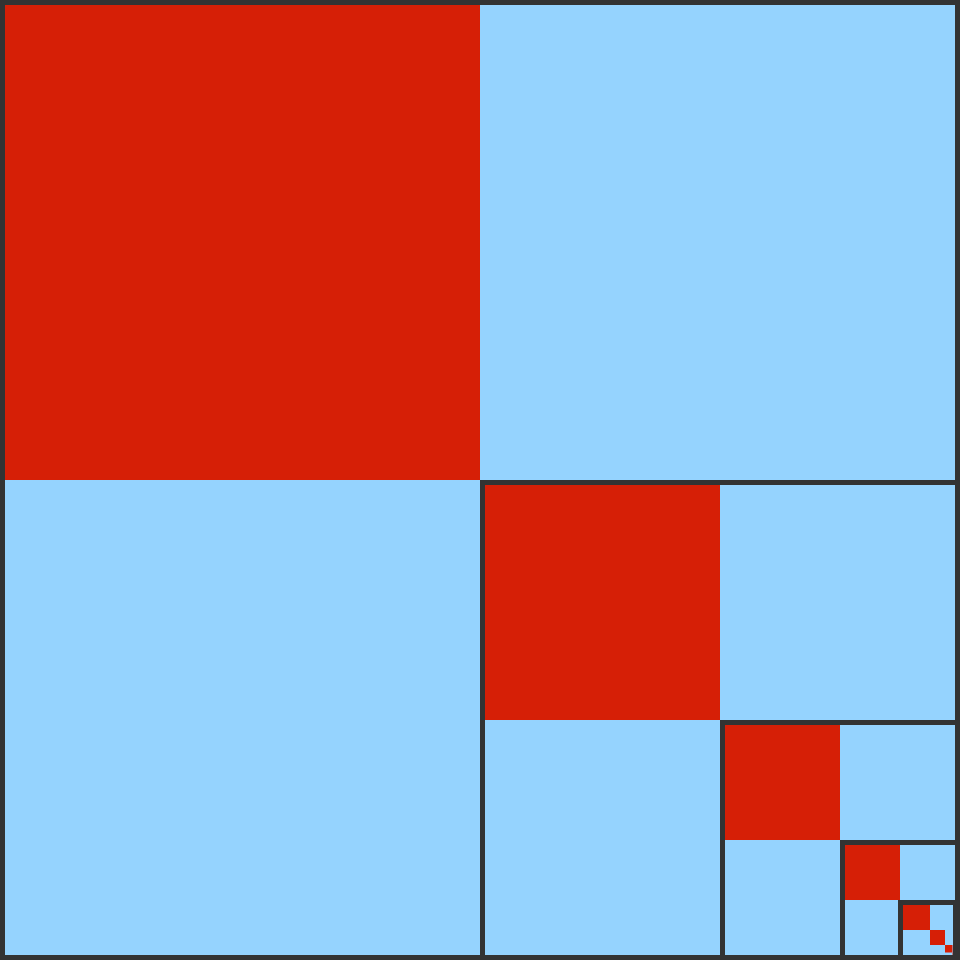In the pattern above, we started with a large blue square, then shaded the top $\frac14$ of it red. Then, in the bottom right quarter, we shaded $\frac14$ of that square red, and so on ad infinitum*.

*Ad infinitum is latin meaning "to infinity".

# Infinite Areas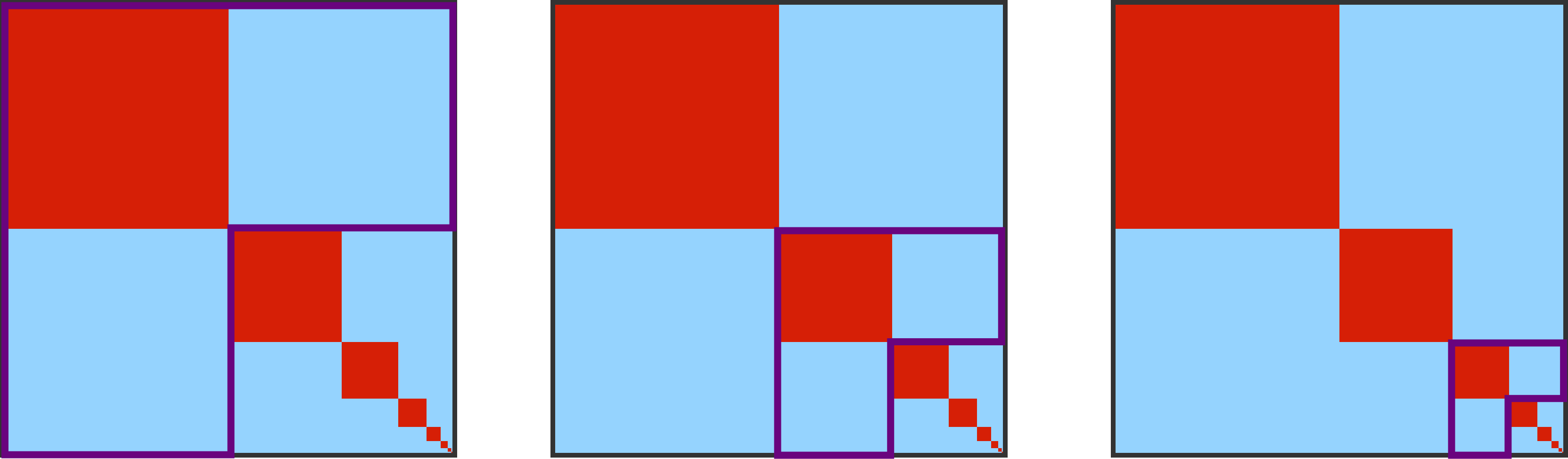In the answer to the prior problem, we found a progressive series of similar L-shapes each with a red portion of $\frac{1}{3},$ and used that to derive the fact that the entire large square has a red portion of $\frac{1}{3} .$

Another approach: take each red square and make two copies, one to the right of the square being copied and one below. The diagram below shows this done in purple.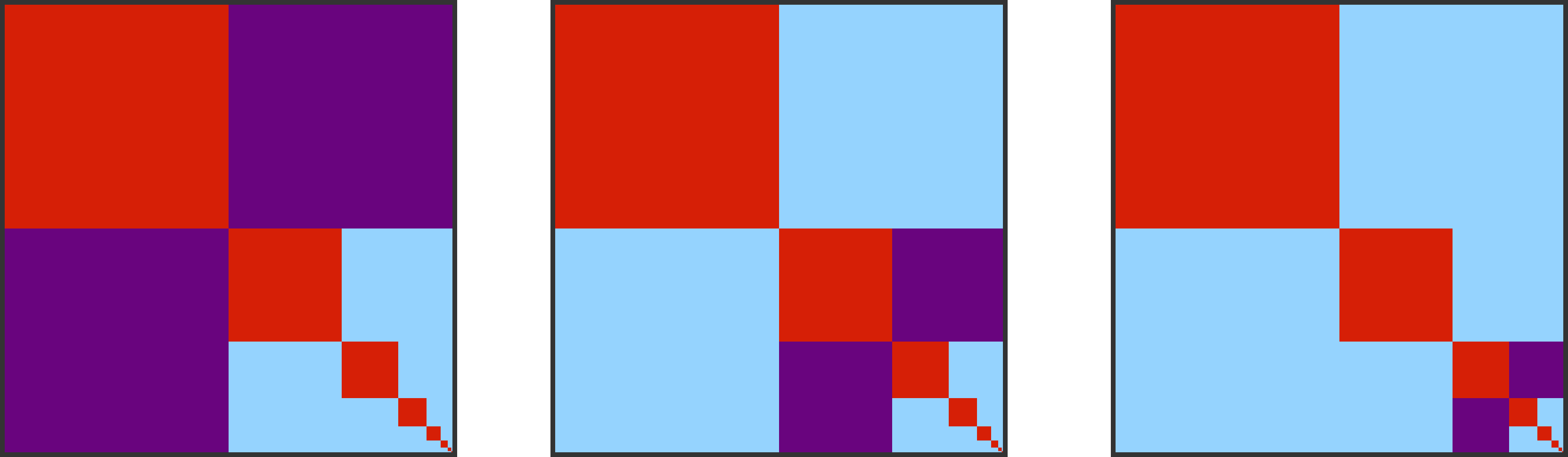This method of covering the large overall square leads to two purple squares for every one red square. This again leads to the red area being $\frac{1}{3}$ of the overall square.

# Infinite Areas

What fraction of this triangle is shaded yellow?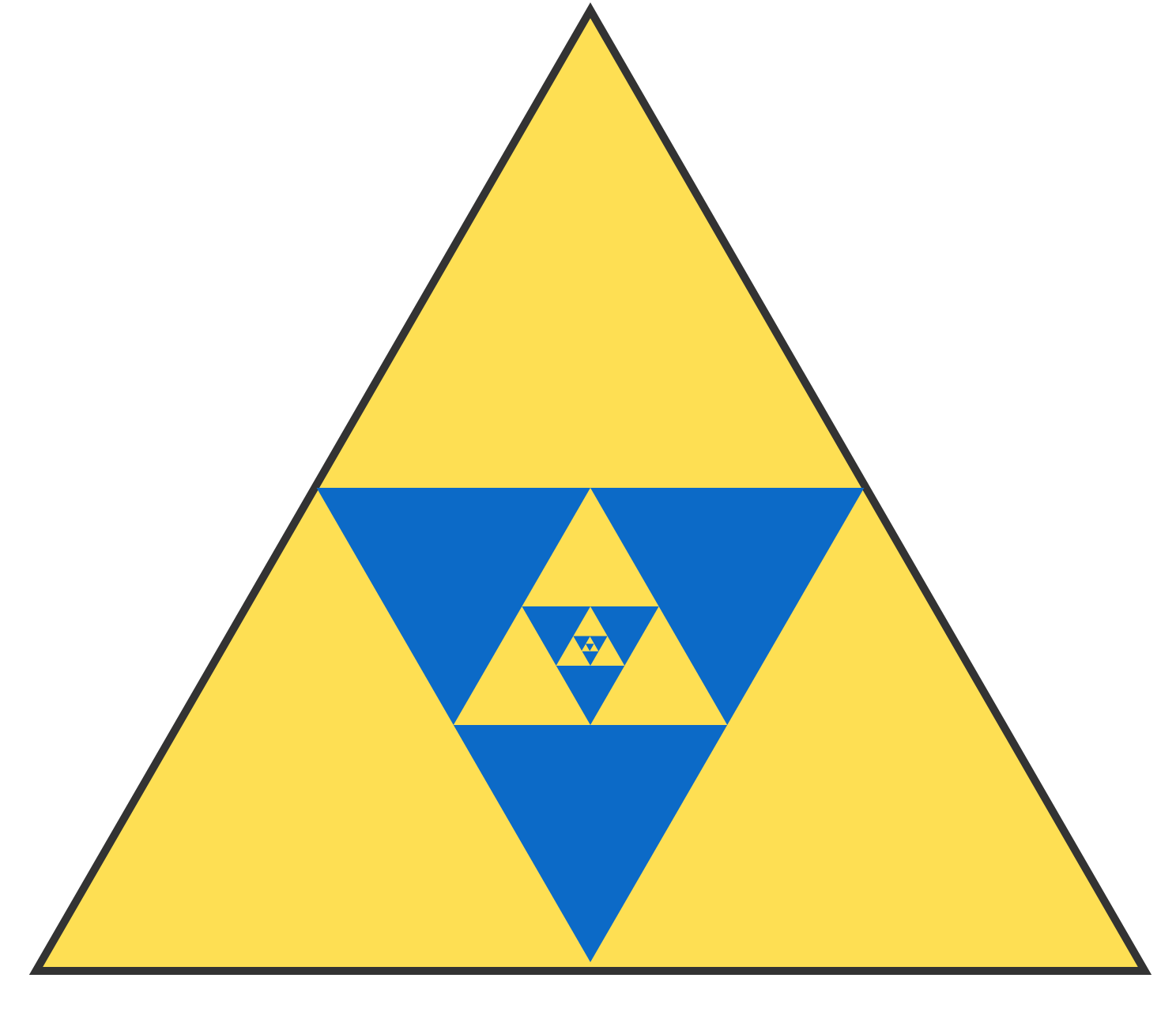Each successively smaller triangle in this pattern connects the midpoints of the three sides of the triangle it's inside of.

# Infinite Areas

How much of this large square is shaded blue?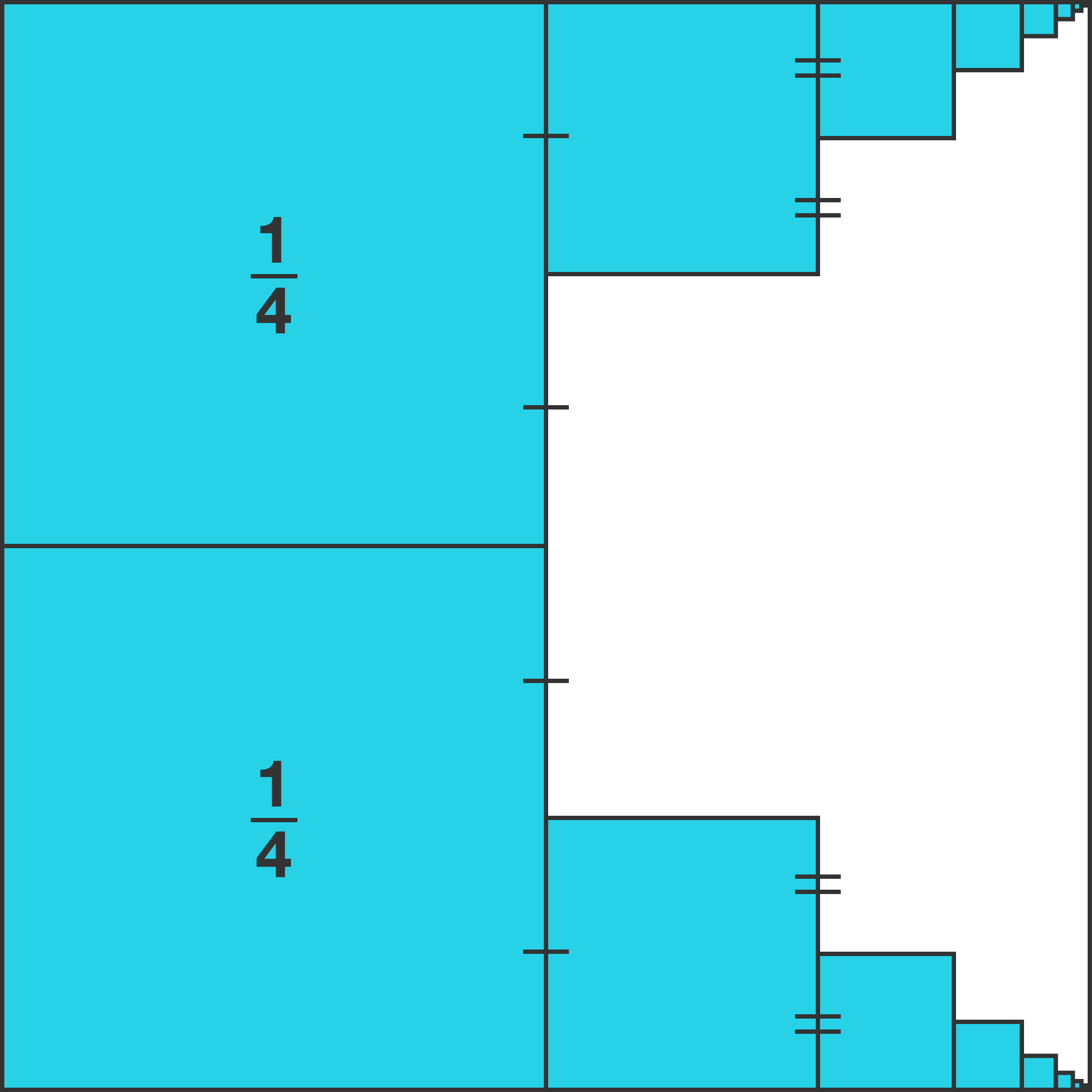To make this figure, first, the left half of a large square was cut into two smaller blue squares each a quarter the area of the original large square. Then, to the right of both squares, there is a succession of blue squares, each one topped by a line that extends from midpoint of the square to the left of it.

# Infinite Areas

What fraction of the largest circle is shaded orange?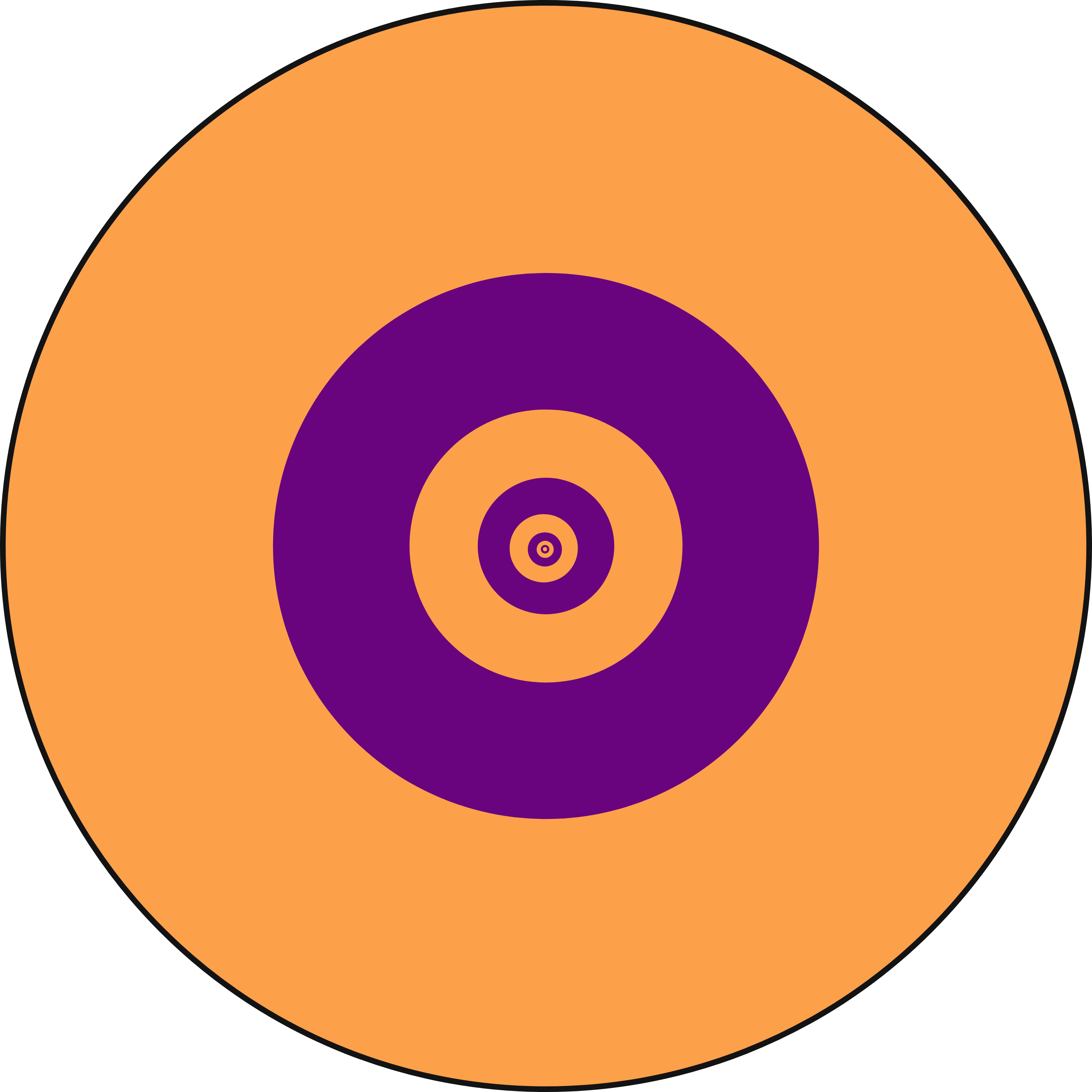All circles in the pattern have the same center, each radius is half the length of the radius of the previous circle, and the color pattern alternates orange-purple-orange-purple-etc.

# Infinite Areas

In the limit as this process is continued ad infinitum, what fraction of the original blue square will be shaded red?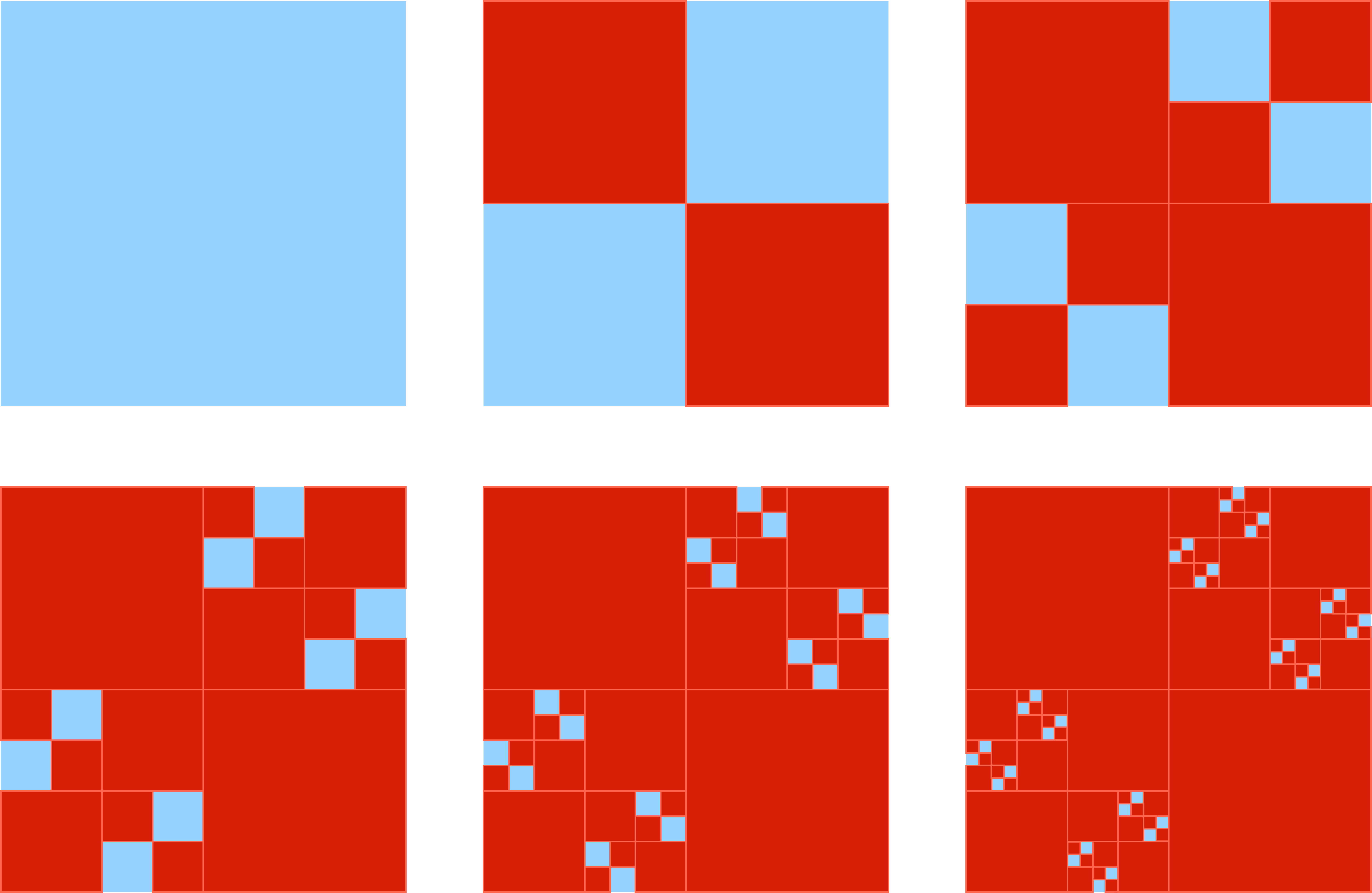Start with a large blue square. Then make 2 red squares, each half the length of the original square, and arrange them diagonally in two opposite corners of the blue square. Then add 4 red squares, each one half the length of the first red squares. Then add 8 red squares, again, making each square half the length of the previous size.

# Infinite Areas

The previous problem could be thought of as a sum of an infinite number of fractions.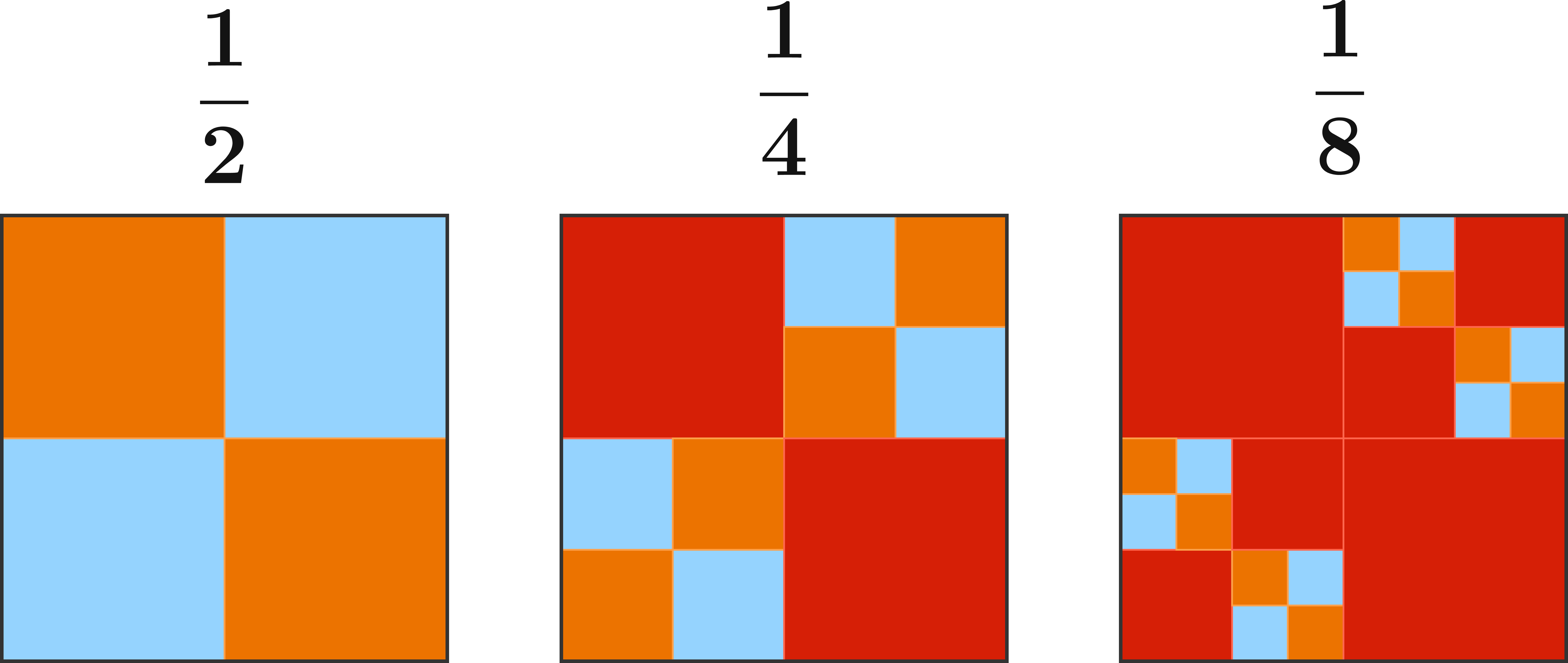$\frac{1}{2} + \frac{1}{4} + \frac{1}{8} + \frac{1}{16} + \cdots$

We'll explore and generalize this useful technique further in this course's tessellations sequence!

×